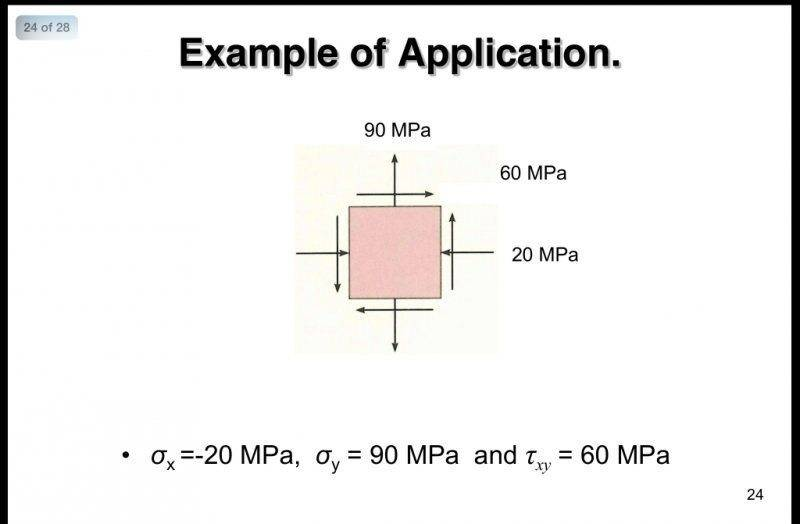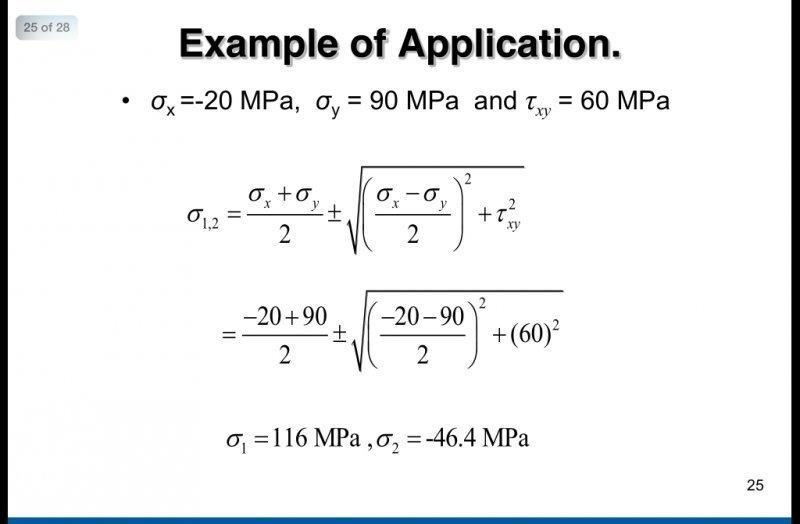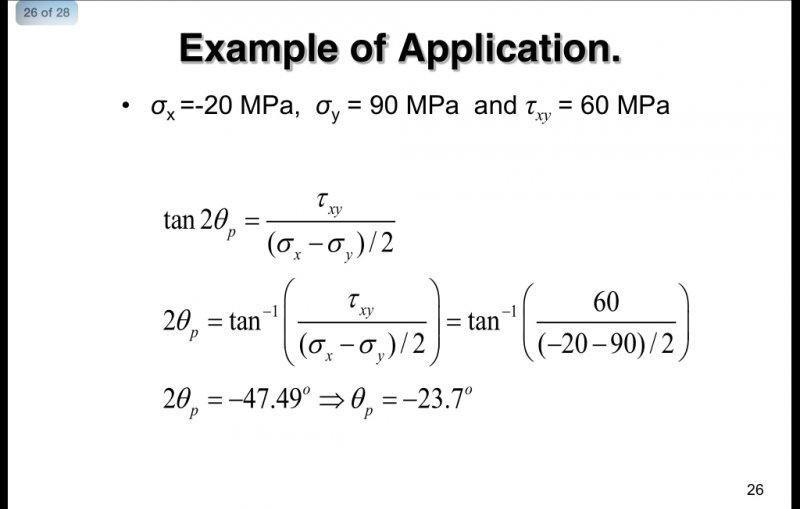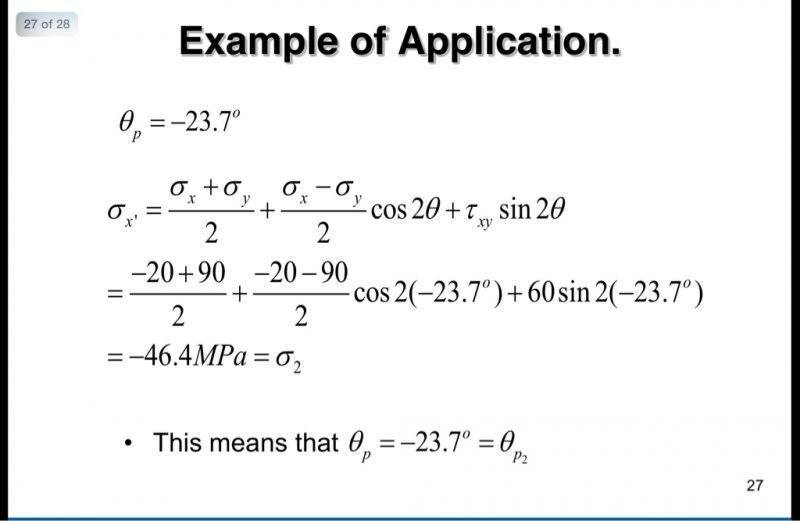# Substituting principal stress angle into the plane stress transformation equationsWhen one substitutes the angle -23.7 into the plane stress transformation equation for SigmaX' you indeed get -46.42 MPa (as shown in the images). However, if you substitute this same angle into the plane stress transformation equation for SigmaY', you yield the other principal stress of 116.4 MPa. My question is how can you conclude the angle -23.7 corresponds to SigmaX' rather than SigmaY' ?

Chestermiller
Mentor
View attachment 81981View attachment 81982View attachment 81983View attachment 81984

When one substitutes the angle -23.7 into the plane stress transformation equation for SigmaX' you indeed get -46.42 MPa (as shown in the images). However, if you substitute this same angle into the plane stress transformation equation for SigmaY', you yield the other principal stress of 116.4 MPa. My question is how can you conclude the angle -23.7 corresponds to SigmaX' rather than SigmaY' ?
The angle θ is the angle that you have to rotate the x axis to get the x' axis.

Chet

•influx
what the difference between angles of theta p and theta which it used to find sigma theta?